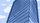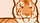cancel
Showing results for
Did you mean:

## (97) This transaction can not be accepted. SIM API Classic ASP

I am trying to start my coding/testing with a basic SIM form. I am getting (97) error.

I am using the simlib.asp and md5.asp files that I downloaded from the barrynovak5/SWOGC
repository to create my timestamp and fingerprint. I have used the timestamp checking
tool ( http://developer.authorize.net/tools/responsecode97/ ) and my result is always
a small positive number < 60. I have taken the code down to bare bones and posted it
below. Any ideas?

<%@LANGUAGE="VBSCRIPT"%>
<% option explicit %>
<!DOCTYPE html PUBLIC "-//W3C//DTD XHTML 1.0 Transitional//EN" "http://www.w3.org/TR/xhtml1/DTD/xhtml1-transitional.dtd">
<html xmlns="http://www.w3.org/1999/xhtml" lang="en" xml:lang="en">
<!--#include virtual="/includes/simlib.asp" -->
<body>

<% dim utcDate, nSeqNumber, cAmount, nLoginId, nTransKey, sFingerprint
nTransKey = "My Transaction Key"
utcDate = simTimeStamp( now() )
cAmount = "12.50"
Dim max,min
max=1000
min=1
Randomize
nSeqNumber = (Int((max-min+1)*Rnd+min))
sFingerprint = HMAC( nTransKey, nLoginId & "^" & nSeqNumber & "^" & utcDate & "^" & cAmount & "^" )
%>
<FORM METHOD=POST ACTION= "https://test.authorize.net/gateway/transact.dll">
<INPUT TYPE=HIDDEN NAME="x_version" VALUE="3.1">
<INPUT TYPE=HIDDEN NAME="x_fp_hash" VALUE="<%=sFingerprint%>">
<INPUT TYPE=HIDDEN NAME="x_fp_sequence" VALUE="<%=nSeqNumber%>">
<INPUT TYPE=HIDDEN NAME="x_fp_ timestamp" VALUE="<%=utcDate%>">
<INPUT TYPE=HIDDEN NAME="x_amount" VALUE="<%=cAmount%>">
<INPUT TYPE=HIDDEN NAME="x_trans_id" VALUE="<%=nTransKey%>">

</FORM>
</body>Member
2 REPLIES 2

Hello @cyberella

I would suggest look at the SIM sample code on this thread.  It's no longer supported by us but it may provide some clues if you compare to the code you have.

Richard